## With Great Power...

Why do we care about power? Power is the measurement of energy transfer over time, and energy costs money. Batteries aren’t free, and neither is that stuff coming out of your electrical outlet. So, power measures how fast the pennies are draining out of your wallet!

Also, energy is…energy. It comes in many, potentially harmful, forms – heat, radiation, sound, nuclear, etc. – ,and more power means more energy. So, it’s important to have an idea of what kind of power you’re working with when playing with electronics. Fortunately, in playing with Arduinos, lighting up LEDs, and spinning small motors, losing track of how much power you’re using only means smoking a resistor or melting an IC. Nevertheless, Uncle Ben’s advice doesn’t just apply to superheros.

#### Covered in this Tutorial

• The definition of power
• Examples of electric energy transfers
• Watts, the SI unit of power
• Calculating power using voltage, current, and resistance
• Maximum power ratings

Power is one of the more fundamental concepts in electronics. But before learning about power, there might be some other tutorials you should read first. If you’re not familiar with some these topics, consider checking out those tutorials first:

## What is Electric Power?

There are many types of power – physical, social, super, odor blockinglove – but in this tutorial, we’ll be focusing on electric power. So what is electric power?

In general physics terms, power is defined as the rate at which energy is transferred (or transformed).

So, first, what is energy and how is it transferred? It’s hard to state simply, but energy is basically the ability ofsomething to move something else. There are many forms of energy: mechanical, electrical, chemical, electromagnetic, thermal, and many others.

Energy can never be created or destroyed, only transferred to another form. A lot of what we’re doing in electronics is converting different forms of energy to and from electric energy. Lighting LEDs turns electric energy into electromagnetic energy. Spinning motors turns electric energy into mechanical energy. Buzzing buzzers makes sound energy. Powering a circuit off a 9V alkaline battery turn chemical energy into electrical energy. All of these are forms ofenergy transfers.

Energy type converted Converted by
Mechanical Electric Motor
Electromagnetic LED
Heat Resistor
Chemical Battery
Wind Windmill

Example electric components, which transfer electric energy to another form.

Electric energy in particular, begins as electric potential energy – what we lovingly refer to as voltage. When electrons flow through that potential energy, it turns into electric energy. In most useful circuits, that electric energy transforms into some other form of energy. Electric power is measured by combining both how much electric energy is transferred, and how fast that transfer happens.

#### Producers and Consumers

Each component in a circuit either consumes or produces electric energy. A consumer transforms electric energy into another form. For example, when an LED lights up, electric energy is transformed into electromagnetic. In this case, the lightbulb consumes power. Electric power is produced when energy is transferred to electric from some other form. A battery supplying power to a circuit is an example of a power producer.

## Wattage

Energy is measured in terms of joules (J). Since power is a measure of energy over a set amount of time, we can measure it in joules per second. The SI unit for joules per second is the watt abbreviated as W.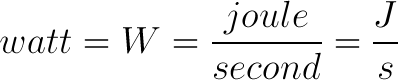It’s very common to see “watts” preceded by one of the standard SI prefixes: microwatts (µW), miliwatt (mW), kilowatt (kW), megawatt (MW), and gigawatts (GW), are all common depending on the situation.

Prefix Name Prefix Abbreviation Weight
Nanowatt nW 10-9
Microwatt µW 10-6
Milliwatt mW 10-3
Watt W 100
Kilowatt kW 103
Megawatt MW 106
Gigawatt GW 109

Microcontrollers, like the Arduino will usually operate in the the µW or mW range. Laptop and desktop computers operate in the standard watt power range. Energy consumption of a house is usually in the kilowatt range. Large stadiums might operate at the megawatt scale. And gigawatts come into play for large-scale power stations and time machines.

## Calculating Power

Electric power is the rate at which energy is transferred. It’s measured in terms of joules per second (J/s) – a watt (W). Given the few basic electricity terms we know, how could we calculate power in a circuit? Well, we’ve got a very standard measurement involving potential energy – volts (V) – which are defined in terms of joules per unit of charge (coulomb) (J/C). Current, another of our favorite electricity terms, measures charge flow over time in terms of the ampere (A) – coulombs per second (C/s). Put the two together and what do we get?! Power!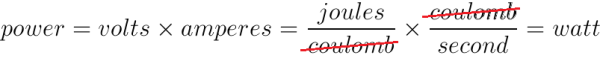To calculate the power of any particular component in a circuit, multiply the voltage drop across it by the current running through it.#### For Example

Below is a simple (though not all that functional) circuit: a 9V battery connected across a 10Ω resistor.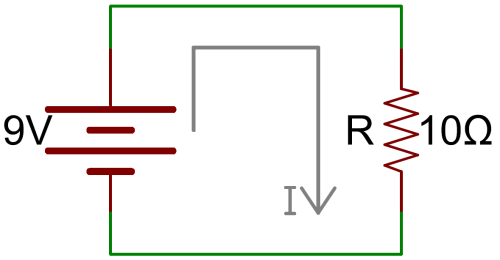How do we calculate the power across the resistor? First we have to find the current running through it. Easy enough…Ohm’s law!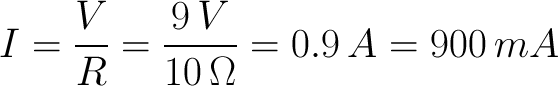Alright, 900mA (0.9A) running through the resistor, and 9V across it. What kind of power is being applied to the resistor then?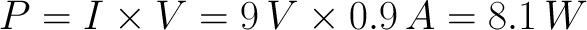A resistor transforms electric energy into heat. So this circuit transforms 8.1 joules of electric energy to heat every second.

#### Calculating Power in Resistive Circuits

When it comes to calculating power in a purely resistive circuit, knowing two of three values (voltage, current, and/or resistance) is all you really need.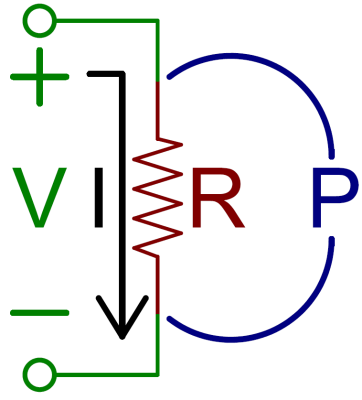By plugging Ohm’s law (V=IR or I=V/R) into our traditional power equation we can create two new equations. The first, purely in terms of voltage and resistance: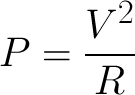So, in our previous example, 9V2/10Ω (V2/R) is 8.1W, and we never have to calculate the current running through the resistor.

A second power equation can be formed solely in terms of current and resistance: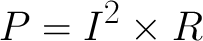Why do we care about the power dropped on a resistor? Or any other component for that matter. Remember that power is the transfer of energy from one type to another. When that electrical energy running from the power source hits the resistor, the energy transforms into heat. Possibly more heat than the resistor can handle. Which leads us to…power ratings.

## Power Ratings

All electronic components transfer energy from one type to another. Some energy transfers are desired: LEDs emitting light, motors spinning, batteries charging. Other energy transfers are undesirable, but also unavoidable. These unwanted energy transfers are power losses, which usually show up in the form of heat. Too much power loss – too much heat on a component – can become very undesirable.

Even when energy transfers are the main goal of a component, there’ll still be losses to other forms of energy. LEDs and motors, for example, will still produce heat as a byproduct of their other energy transfers.

Most components have a rating for maximum power they can dissipate, and it’s important to keep them operating under that value. This’ll help you avoid what we lovingly refer to as “letting the magic smoke out”.

#### Resistor Power Ratings

Resistors are some of the more notorious culprits of power loss. When you drop some voltage across a resistor, you’re also going to induce current flow across it. More voltage, means more current, means more power.

Remember back to our first power-calculation example, where we found that if 9V were dropped across a 10Ω resistor, that resistor would dissipate 8.1W. 8.1 is a lot of watts for most resistors. Most resistors are rated for anywhere from ⅛W (0.125W) to ½W (0.5W). If you drop 8W across a standard ½W resistor, ready a fire extinguisher.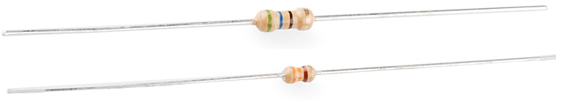If you’ve seen resistors before, you’ve probably seen these. Top is a ½W resistor and below that a ¼W. These aren’t built to dissipate very much power.

There are resistors built to handle large power drops. These are specifically called out as power resistors.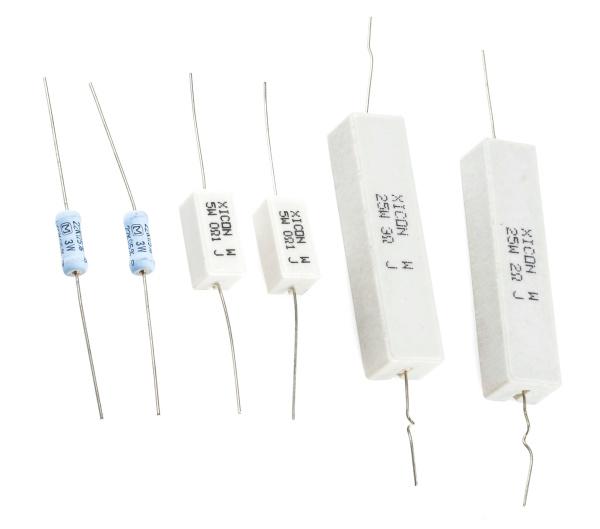These large resistors are built to dissipate lots of power. From left to right: two 3W 22kΩ resistors, two 5W 0.1Ω resistors, and 25W 3Ω and 2Ω resistors.

If you ever find yourself picking out a resistor value. Keep it’s power rating in mind as well. And, unless your goal is to heat something up (heating elements are basically really high-power resistors), try to minimize power loss in a resistor.

##### For Example

Resistor power ratings can come into play when you’re trying to decide on a value for an LED current-limiting resistor. Say, for example, you want to light up a 10mm super-bright red LED at maximum brightness, using a 9V battery.That LED has a maximum forward current of 80mA, and a forward voltage of about 2.2V. So to deliver 80mA to the LED, you’d need an 85Ω resistor to do so.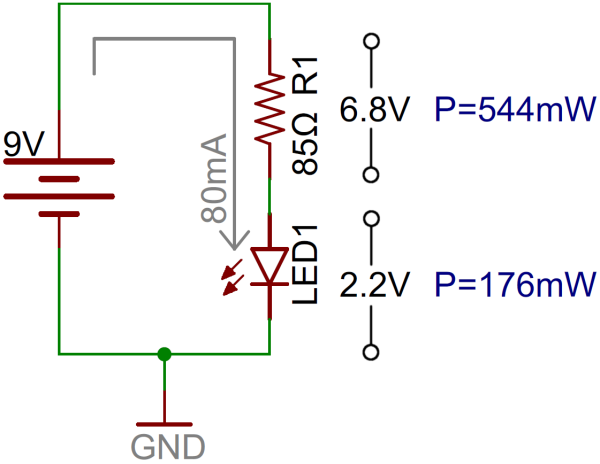6.8V dropped on the resistor, and 80mA running through it means 0.544W (6.8V*0.08A) of power lost on it. A half-watt resistor isn’t going to like that very much! It probably won’t melt, but it’ll get hot. Play it safe and move up to a 1W resistor (or save power and use a dedicated LED driver).

Resistors certainly aren’t the only components where maximum power ratings must be considered. Any component with a resistive property to it is going to produce thermal power losses. Working with components that are commonly subjected to high power – voltage regulators, diodes, amplifiers, and motor drivers, for example – means paying extra special attention to power loss and thermal stress.

## Resources and Going Further

Now that you’re familiar with the concept of electric power, check out some of these related tutorials!

• How to Power Your Project - Well, you know what “power” is. But how do you get it to your project?
• Light - Light is a useful tool for the electrical engineer. Understanding how light relates to electronics is a fundamental skill for many projects.
• What is an Arduino - We talked about this Arduino thing a lot in this tutorial. If you’re still unclear about what that is, check this tutorial out!
• Diodes - Whether they’re converting AC to DC, or just lighting up a power indicator LED, diodes are an especially handy component for powering projects.
• Resistors - The most basic of electronic components, resistors are a requirement in just about every circuit there is.
• MP3 Player Shield Music Box - Talk about energy transfer! This project combines electricity, motion, and sound to make a Doctor Who-themed music box.